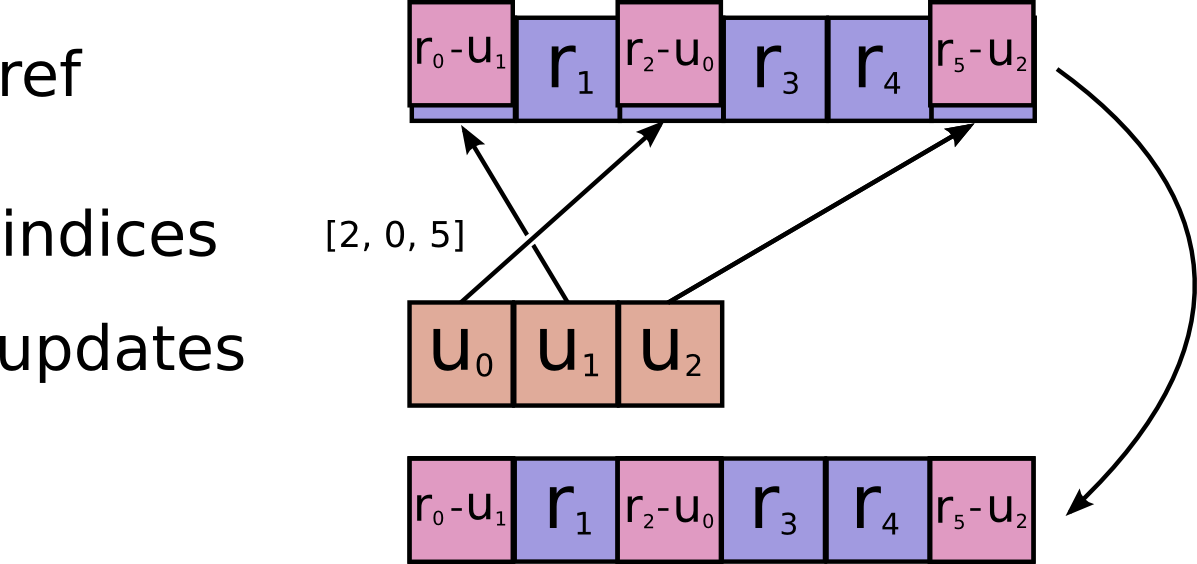# tf.compat.v1.scatter_sub

Subtracts sparse updates to a variable reference.

``````    # Scalar indices

# Vector indices (for each i)

# High rank indices (for each i, ..., j)
ref[indices[i, ..., j], ...] -= updates[i, ..., j, ...]
``````

This operation outputs `ref` after the update is done. This makes it easier to chain operations that need to use the reset value.

Duplicate entries are handled correctly: if multiple `indices` reference the same location, their (negated) contributions add.

Requires `updates.shape = indices.shape + ref.shape[1:]` or `updates.shape = []`.`ref` A mutable `Tensor`. Must be one of the following types: `float32`, `float64`, `int32`, `uint8`, `int16`, `int8`, `complex64`, `int64`, `qint8`, `quint8`, `qint32`, `bfloat16`, `uint16`, `complex128`, `half`, `uint32`, `uint64`. Should be from a `Variable` node.
`indices` A `Tensor`. Must be one of the following types: `int32`, `int64`. A tensor of indices into the first dimension of `ref`.
`updates` A `Tensor`. Must have the same type as `ref`. A tensor of updated values to subtract from `ref`.
`use_locking` An optional `bool`. Defaults to `False`. If True, the subtraction will be protected by a lock; otherwise the behavior is undefined, but may exhibit less contention.
`name` A name for the operation (optional).

A mutable `Tensor`. Has the same type as `ref`.

[]
[]